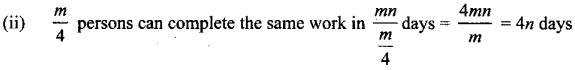Tamilnadu State Board New Syllabus Samacheer Kalvi 8th Maths Guide Pdf Chapter 4 Life Mathematics InText Questions Text Book Back Questions and Answers, Notes.

## Tamilnadu Samacheer Kalvi 8th Maths Solutions Chapter 4 Life Mathematics InText Questions

Try These (Text Book Page No. 122)

Find the indicated percentage value of the given numbers.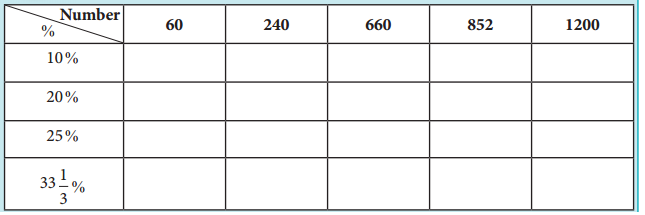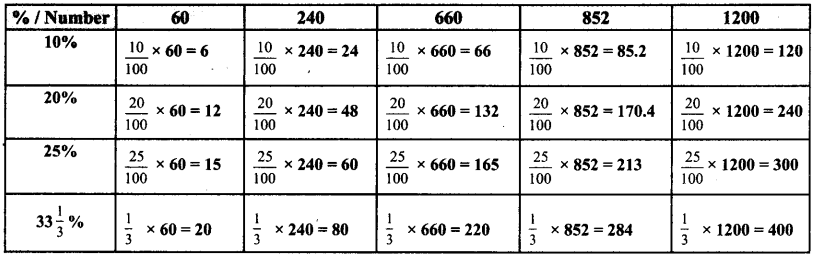Try These (Text Book Page No. 124)

Question 1.
What percentage ola day is 10 hours?
In a day, there are 24 hours .
∴ 10 hrs out of 24 hrs is $$\frac{10}{24}$$
As a percentage, we need to multiply by 100
∴ Percentage = $$\frac{10}{24}$$ × 100 = 41.67%

Question 2.
Divide ₹ 350 among P, Q and R such that P gets 50% of what Q gets and Q gets 50% of what R gets.
Let R get x, Q gets 50% of what R gets
∴ Q gets = $$\frac{50}{100} \times x=\frac{x}{2}$$
P gets 50% of what Q gets .
∴ P gets = $$\frac{50}{100} \times \frac{x}{2}=\frac{x}{4}$$
Since 350 is divided among the three
∴ 350 = $$x+\frac{x}{2}+\frac{x}{4}$$
350 = $$\frac{4 x+2 x+x}{4}=\frac{7 x}{4}$$ = 350
x = $$\frac{350 \times 4}{7}$$
Q gets = $$\frac{x}{2}=\frac{200}{2}$$ = 100,
P gets = $$\frac{x}{4}=\frac{200}{4}$$ = 50
∴ p = 50, Q = 100, R = 200Think (Text Book Page No. 124)

With a lot of pride, the traffic police commissioner of a city reported that the accidents had decreased by 200% in one year. He came up with this number by stating that the increase in accidents from 200 to 600 is clearly a 200% rise and now that it had gone down from 600 last year to 200 this year should be a 200% fall. Is this decrease from 600 to 200, the same 200% as reported by him? Justify.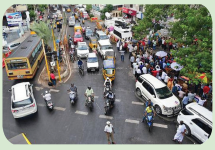Increase from original value 200 to 600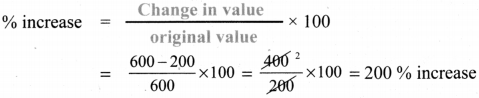Decrease from original value 600 to 200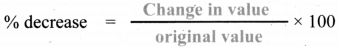here original value is 600
% decrease = $$\frac{600-200}{600}$$ × 100 = $$\frac{400}{600}$$ × 100 = 66.67 % decrease
Increase from 200 → 600 and % decrease from 600 → 200 are not the sameTry These (Text Book Page No. 126)

Question 1.
If the selling price of an article is less than the cost price of the article, then there is a ________ .
Loss

Question 2.
An article costing 5000 is sold for ₹ 4850. Is there a profit or loss? What percentage is it?
Loss
Percentage of Loss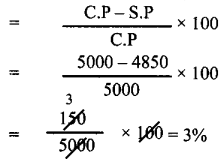Question 3.
If the ratio of cost price and the selling price of an article is 5:7, then the profit / gain is ________ %.
C.P = 5x
S.P = 7x
Profit = 7x – 5x = 2x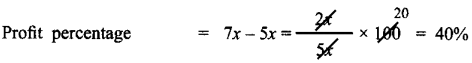Think (Text Book Page No. 129)

A shopkeeper marks the price of a marker board 15% above the cost price and then allows a discount of 15% on the marked price. Does he gain or lose in the transaction?
Let cost price of marker board be 100
CP = 100 Marks it 15% above CP
∴ Marked price MP = $$\frac{15}{100}$$ × CP + CP
= $$\frac{15}{100}$$ × 100 + 100 = 15 + 100 = 115
Discount % = 15 %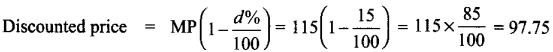∴ He sells it 97.75 which is less than his cost price. Therefore he loses
Loss = 97.75 – 100 = – 2.25Try These (Text Book Page No. 129)

Question 1.
The formula to find the simple interest for a given principal is ________ .
$$\frac{\mathrm{PNR}}{100}$$

Question 2.
Find the simple interest on ₹ 900 for 73 days at 8% p.a.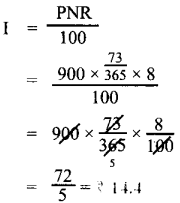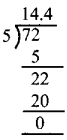Question 3.
In how many years will ₹ 2000 become ₹ 3600 at 10% p.a simple interest?
I = 3600 – 2000 = 1600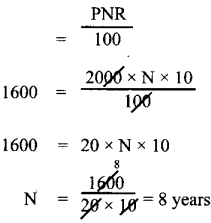Try These (Text Book Page No. 141)

Question 1.
Classify the given examples as direct or inverse proportion:
(i) Weight of pulses to their cost.
As weight increases cost also increases.
∴ Weight and cost are direct proportion.

(ii) Distance travelled by bus to the price of ticket.
As the distance increases price to travel also increases,
∴ Distance and price are direct proportion.(iii) Speed of the athelete to cover a certain distance.
As the speed increases, the time to cover the distance become less.
So speed and üme are in indirect proportion.

(iv) Number of workers employed to complete a construction in a specified time.
As the number of workers increases, the amount of work become less, so they are in indirect proportion.

(v) Area of a circle to its radius.
If the radius of the circle increases its area also increases.
∴ Area and radius of circles are direct proportion.Question 2.
A student can type 21 pages in 15 minutes. At the same rate, how long will it take student to type 84 pages?
Direct proportion
No. of minutes = x
k = $$\frac{21}{15}$$
$$\frac{21}{15}=\frac{84}{x}$$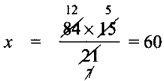Question 3.
If 35 women can do a piece of work in 16 days, In how many days will 28 women do the same work?
Inverse proportion
No. of days = x
k = 35 × 16
∴ 28 × x = 35 × 16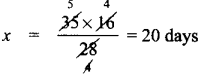Try These (Text Book Page No. 145)

Question 1.
If x and y vary directly, find k when x = y = 5.
I If x andy vary directly then $$\frac{x}{y}$$ = k.
Here x = 5; y = 5
∴ k = $$\frac{5}{5}$$
k = 1

Question 2.
If x and y vary inversely, find the constant of proportionality when x = 64 and y = 0.75
Gìven x = 64, y = 0.75
and also given x andy vary inversely.
∴ xy = k. the constant of variation.
∴ Constant = 64 × 0.75
Constant of variation = 48Activity (Text Book Page No. 145)

Draw a circle of a given radius. Then, draw its radii in such a way that the angles between any two consecutive pair of radii are equal. Start drawing 3 radii and end with drawing 12 radii in the circle. List and prepare a table for the number of radii to the angle between a pair of consecutive radii and check whether they are in inverse proportion. What is the proportionality constant?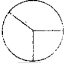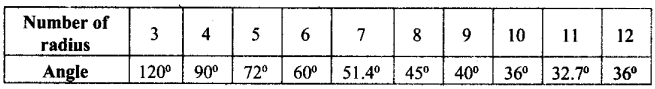As the number of radii increases angle decreases.
Hence they are in inverse proportion
∴ xy = 4 proportional constant
3 × 120° = 360° = k = 360°Try These (Text Book Page No. 147)

Identify the different variations present in the following questions:

Question 1.
24 men can make 48 articles in 12 days. Then, 6 men can make _____ articles in 6 days.
Let the required no. of articles be x

 Men (P) Days (D) Articles (W) 24 12 48 6 6 x

(i) Mens and days are Indirect variables.
(ii) Men and Articles are direct vanables
(iii) Days and articles are also direct variables using formula.
Let
P1 = 24
P2 = 6

D1 = 12
D2 = 6

W1 = 48
W1 = x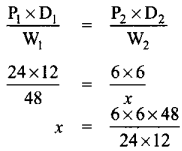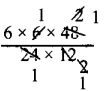x = 6 ArticlesQuestion 2.
15 workers can lay a road of length 4 km in 4 hours. Then, _____ workers can lay a road of length 8 km in 8 hours.
Let the required no. of workers be x

 Length (work) Hours Workers 4 km 4 hrs 15 8 km 8 hrs x

(i) Length and workers are direct variable as more length need more workers.
The proportion is 4 : 8 : : 15 : x ——– (1)
(ü) Hours and workers are indirect variables as more working hours need less men.
∴ The proportion is : 4 : : 15 : x ——– (2)
Combining (1) and (2)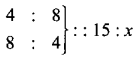Product of the extremes = Product of the mean
4 × 8 × x = 8 × 4 × 15
x = $$\frac{8 \times 4 \times 15}{4 \times 8}$$
x = 15 workersQuestion 3.
25 women working 12 hours a day can complete a work in 36 days. Then, 20 women must work hours a day to complete the same work in 30 days.
Let the required hours be x.

 Women Days Hours 25 36 12 20 30 x

As women increases hours to work decreases
∴ It is an inverse proportion.
∴ Multiplying factor is $$\frac{25}{20}$$
As days increases hours needed become less
∴ It is also an indirect variation.
∴ Multiplying factor is $$\frac{36}{30}$$
∴ x = $$12 \times \frac{25}{20} \times \frac{36}{30}$$
x = 18 hours

Question 4.
In a camp there are 420 kg of rice sufficient for 98 persons for 45 days. The number of days that 60 kg of rice will last for 42 persons is .
Let the required number of days be x.

 Rice (kg) Men Days 420 98 45 60 42 x

If amount of rice is more it will last for more days;
∴ It is Direct Proportion
∴ Multiplying factor is $$\frac{60}{420}$$
If men increases number of days the rice lasts decreases
∴ It is an inverse proportion.
∴ Multiplying factor is $$\frac{98}{42}$$
x = $$45 \times \frac{60}{420} \times \frac{98}{42}$$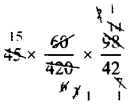x = 15 daysTry These (Text Book Page No. 150)

Question 1.
Vikram can do one-third of work in p days. He can do th of work In ________ days.
$$\frac{1}{3}$$ of the work will be done mp days.
∴ Full work will be completed in 3p days
$$\frac{3}{4}$$ th of the work will be done in = 3p x $$\frac{3}{4}$$
= $$\frac{9}{4}$$p = 2$$\frac{1}{4}$$ p days.

Question 2.
If m persons can complete a work in n days, then 4m persons can complete the same
work in ______ days and $$\frac{m}{4}$$ persons can complete the same work in ______ days.
4 m men do the work it will be completed in $$\frac{m n}{4 m}$$ days = $$\frac{m}{4}$$ days.